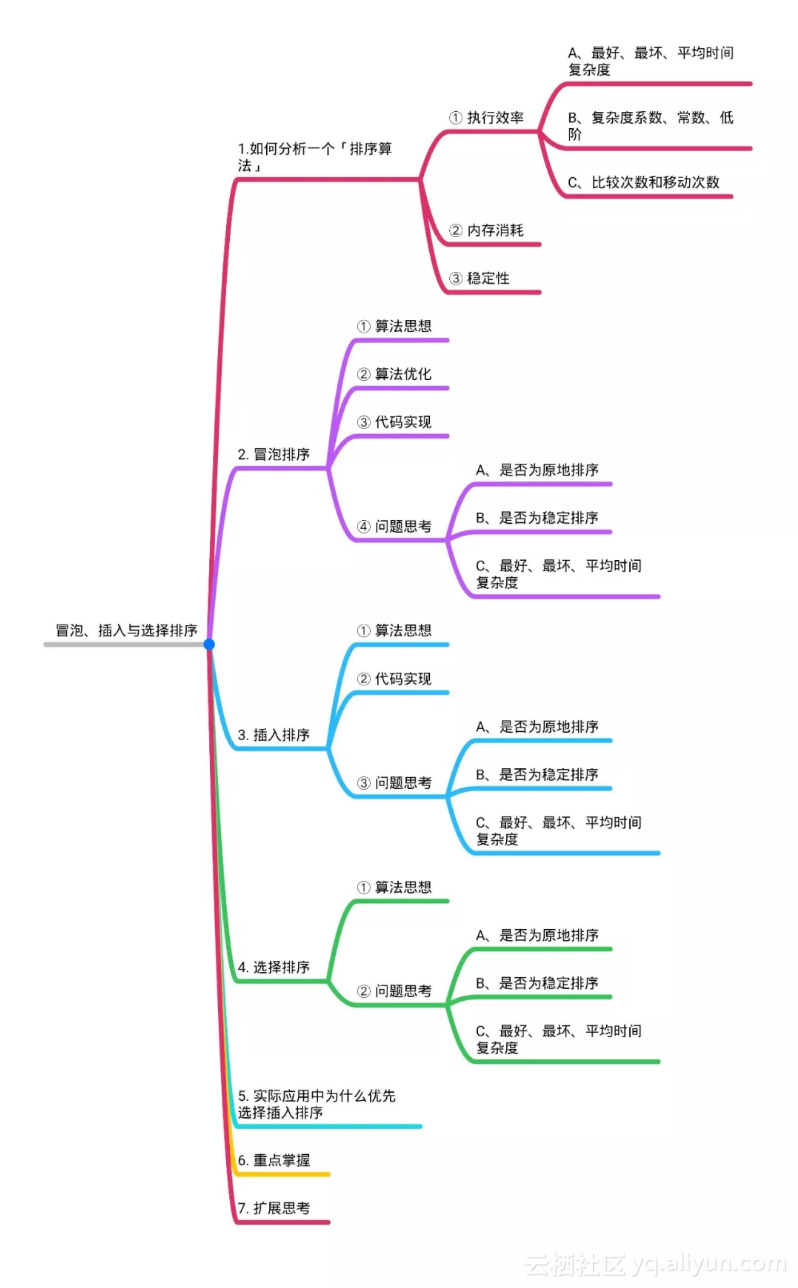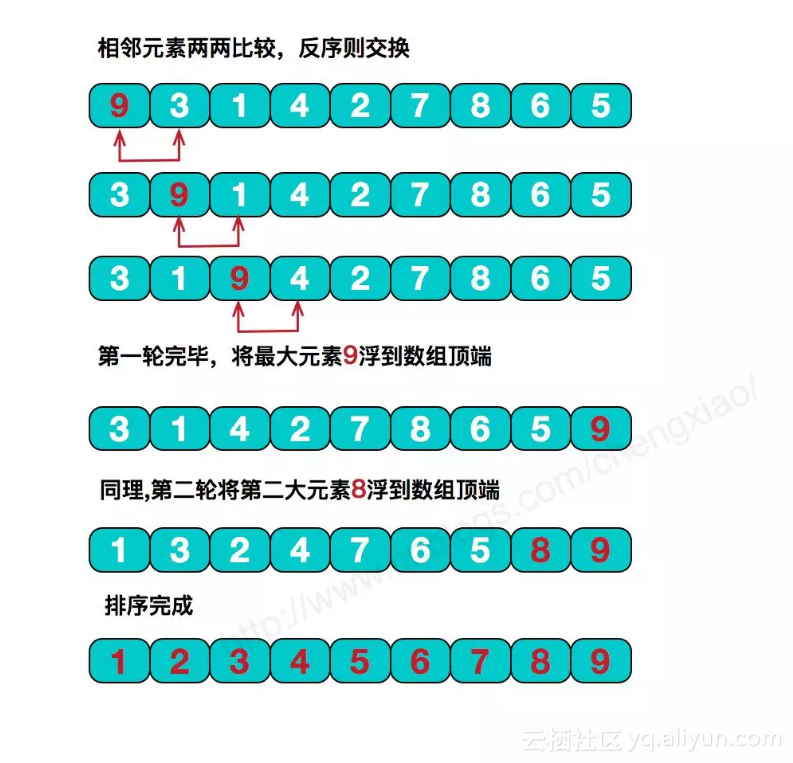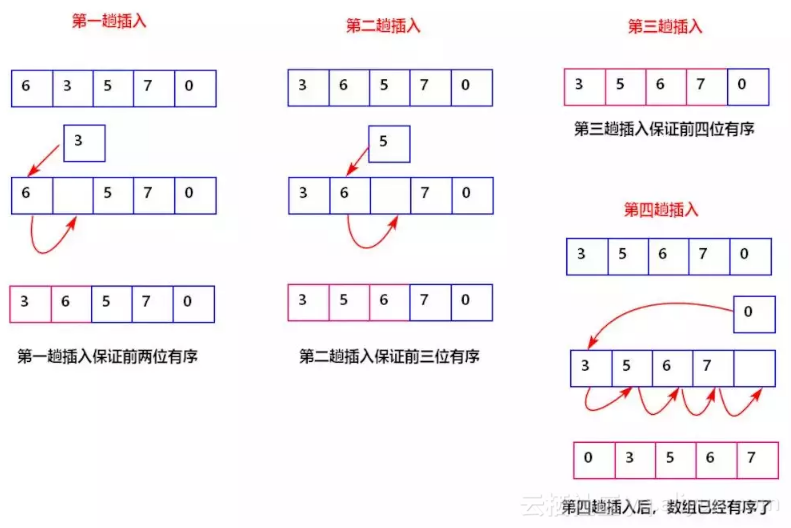0
4
01. 云栖社区>
2. web项目聚集地>
3. 博客>
4. 正文

面试前你必须知道的三个排序算法1. 执行效率

① 最好、最坏、平均时间复杂度

② 复杂度系数、常数、低阶

③ 比较次数和移动次数

2. 内存消耗

3. 稳定性

1、算法思想2、算法优化

3、代码实现

1// 冒泡排序，a 表示数组，n 表示数组大小
2public void bubbleSort(int[] a, int n) {
3 if (n <= 1) return;
4
5 for (int i = 0; i < n; ++i) {
6 // 提前退出冒泡循环的标志位
7 boolean flag = false;
8 for (int j = 0; j < n - i - 1; ++j) {
9 if (a[j] > a[j+1]) { // 交换
10 int tmp = a[j];
11 a[j] = a[j+1];
12 a[j+1] = tmp;
13 flag = true; // 表示有数据交换
14 }
15 }
16 if (!flag) break; // 没有数据交换，提前退出
17 }
18}

4、问题思考

①是否为原地排序

②是否为稳定排序

③最好、最坏以及平均时间复杂度

1、算法思想2、代码实现

1// 插入排序，a 表示数组，n 表示数组大小（从小到大进行排序）
2public void insertionSort(int[] a, int n) {
3 //如果数组大小为 1 直接返回
4 if (n <= 1) return;
5 //否则进行插入排序
6 for (int i = 1; i < n; ++i) {
7 int value = a[i];
8 int j = i - 1;
9 // 查找插入的位置
10 for (; j >= 0; --j) {
11 if (a[j] > value) {
12 a[j+1] = a[j]; // 数据移动
13 } else {
14 break;
15 }
16 }
17 a[j+1] = value; // 插入数据
18 }
19}

3、问题思考

① 是否为原地排序？

② 是否为稳定排序？

③ 最好、最坏、平均时间复杂度？

1、算法思想

2、问题思考

① 是否为原地排序

② 是否为稳定排序

③ 最好、最坏以及平均时间复杂度

3、代码实现

1// 选择排序，a表示数组，n表示数组大小
2 public static void selectionSort(int[] a, int n) {
3 if (n <= 1) return;
4 for (int i = 0; i < n; ++i) {
5 // 查找最小值
6 int minIndex = i;
7 int minValue = a[i];
8 for (int j = i; j < n; ++j) {
9 if (a[j] < minValue) {
10 minValue = a[j];
11 for (int i = 0; i < n - 1; ++i) {
12 // 查找最小值
13 int minIndex = i;
14 for (int j = i + 1; j < n; ++j) {
15 if (a[j] < a[minIndex]) {
16 minIndex = j;
17 }
18 }
19 if (minIndex == i)
20 continue;
21 // 交换
22 int tmp = a[i];
23 a[i] = a[minIndex];
24 a[minIndex] = tmp;
25 }
26 }

1冒泡排序中数据的交换操作：
2if (a[j] > a[j+1]) { // 交换
3 int tmp = a[j];
4 a[j] = a[j+1];
5 a[j+1] = tmp;
6 flag = true;
7}
8
9插入排序中数据的移动操作：
10if (a[j] > value) {
11 a[j+1] = a[j]; // 数据移动
12} else {
13 break;
14}

+ 关注